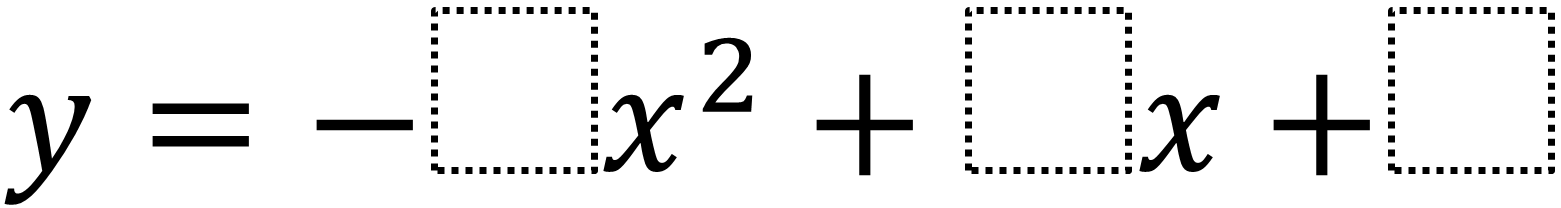# Maximum Value of a Quadratic in Standard Form

Directions: Using the digits 1 to 9 at most one time each, fill in the boxes to create a quadratic equation with the greatest possible maximum value.### Hint

Where is the best place to put the 9? How does each box affect the graph’s behavior?

The quadratic with the greatest possible maximum value is -x^2 + 9x + 8.  Play with the sliders on this Desmos graph for a hands on way of understanding how the a, b, and c terms affect the quadratic: https://www.desmos.com/calculator/kdsj3ic0gn

Source: Robert Kaplinsky

## Period of Trig Function 3

Directions: Using the digits 0 to 9, at most one time, fill in the blanks …

### One comment

1.Paul Muckerheide

If x^2-d^2 =0 is known to your student to have solution plus or minus d for solution and horizontal slide is known to your student then when your student slides the quadratic so axis of symmetry is “new” y axis will your student be able to find zeroes real or non real?
Specific f(x)=x^2-2x-3 slide to f(x+1)=x^2-4. Is this not “easy” to solve. Will your student know how to move (-2,0) back to original x cross y?
Can your student find and prove the quadratic formula from this connection of ideas?